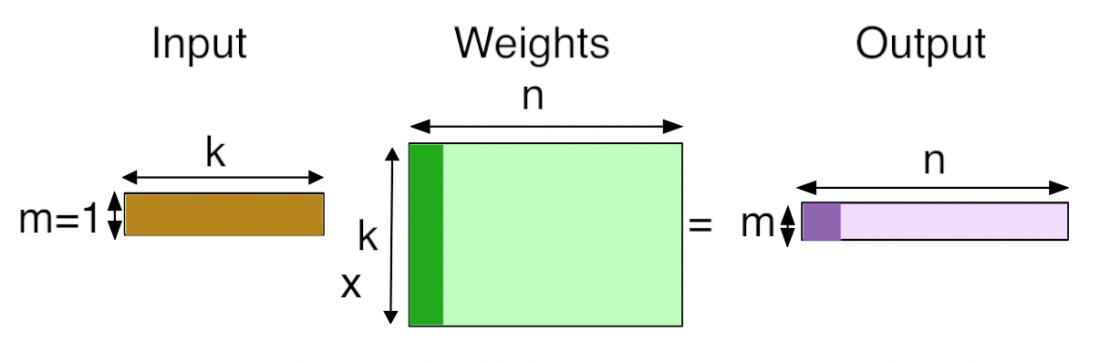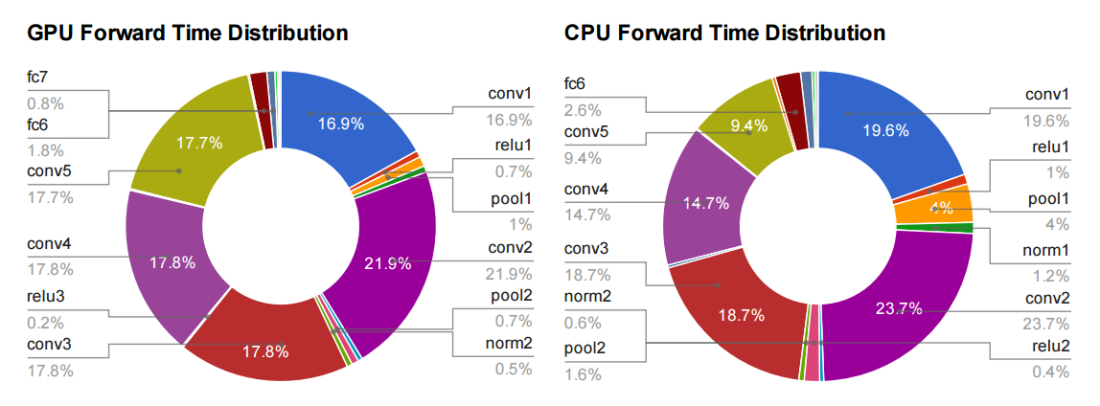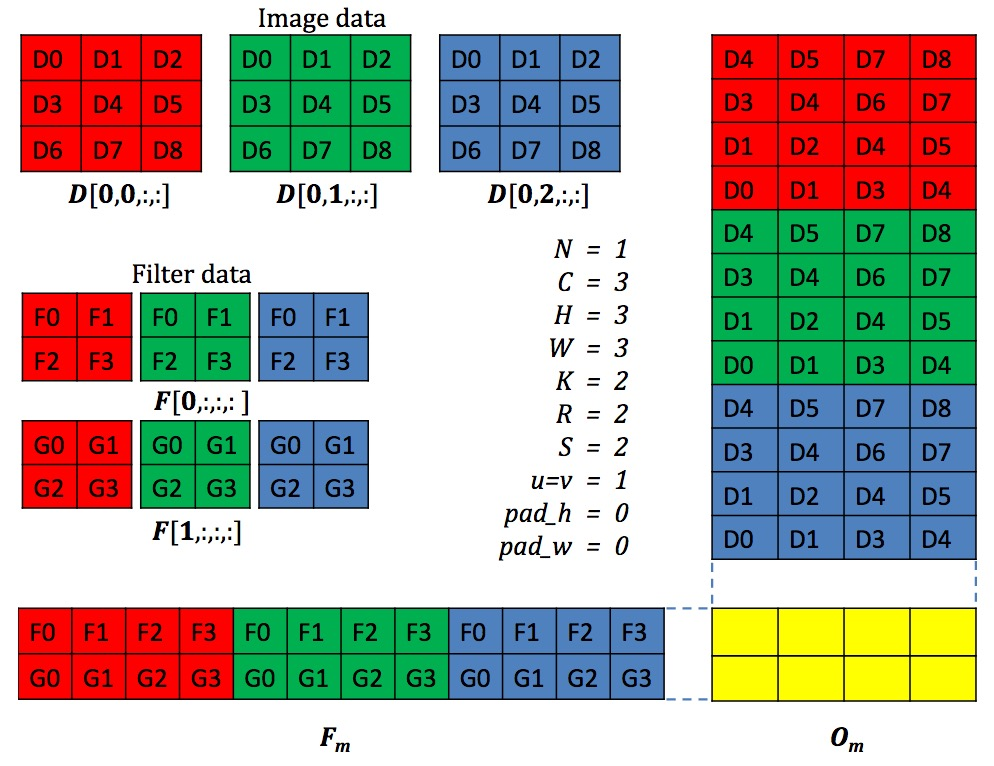## Inner Product Layer

Inner Product Layer即全连接层，对于IP层的理解，可以简单的将其视为矩阵1*N和矩阵N*M相乘后得到1*M的维度向量。

N = 9408
M = num_ouput = 4096layer {
name: "ip1"
type: "InnerProduct"
bottom: "pool2"
top: "ip1"
# learning rate and decay multipliers for the weights
param {
lr_mult: 1
}
# learning rate and decay multipliers for the biases
param {
lr_mult: 2
}
inner_product_param {
num_output: 500
weight_filler {
type: "xavier"
}
bias_filler {
type: "constant"
}
}
}


template <typename Dtype>
void InnerProductLayer<Dtype>::Forward_cpu(const vector<Blob<Dtype>*>& bottom,
const vector<Blob<Dtype>*>& top) {
const Dtype* bottom_data = bottom->cpu_data();
Dtype* top_data = top->mutable_cpu_data();
const Dtype* weight = this->blobs_->cpu_data();
caffe_cpu_gemm<Dtype>(CblasNoTrans, transpose_ ? CblasNoTrans : CblasTrans,
M_, N_, K_, (Dtype)1.,
bottom_data, weight, (Dtype)0., top_data);
if (bias_term_) {
caffe_cpu_gemm<Dtype>(CblasNoTrans, CblasNoTrans, M_, N_, 1, (Dtype)1.,
bias_multiplier_.cpu_data(),
this->blobs_->cpu_data(), (Dtype)1., top_data);
}
}


$\begin{equation} C=alpha*TransA(A)*TransB(B) + beta*C\\\\ \end{equation}$

$\begin{equation} C=alpha*TransA(A)*y + beta*y\\\\ \end{equation}$

## GEMM

GEMM是BLAS (Basic Linear Algebra Subprograms)库的一部分，该库在1979年首次创建。为什么GEMM在深度学习中如此重要呢？我们可以先来看一个图：